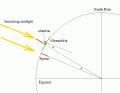# Summer Solstice coming up ... chance to calculate earth's circumference

#### drc_567

Joined Dec 29, 2008
1,156
On June 20 at 4:44 CDT in the northern hemisphere the sun will be vertically overhead at a geographic location in the northern hemisphere. If you are located precisely on a longitudinal line North of that 'zenith position' at some arbitrary northern latitude, you will have the opportunity to calculate the approximate circumference of the earth, assuming that it is spherically shaped.
...The two key numbers that are necessary are the angle that the shadow of a vertical pole or stick forms at precise!y 4:44 CDT, and also the geographic distance between the po!e location and the overhead sun zenith position, which is necessarily located further south of the pole/stick location.
The method was first described by the Greek mathematician Erathosthenes:
Erathosthenes.
An explanatory diagram:Consequently, R, the radius of the earth, is found to be approximately = d/(tan A),
and the circumference C=2π R.
Where:
d is the longitudinal geographic distance between the two points.
A is the angle made by the shadow of the pole at the northern geographic position.

... It may be that this method would be more accurate when a shorter d measurement is possible, as opposed to a vertical stick positioned at an extreme northern latitude. The southern zenith position for my particular location is at approximately 23 degrees North latitude, and my shadow angle measurement position is at about 30 degrees North latitude. I seem to be located on about the same longitudinal line as the sun zenith position, thereby allowing a reasonably simple estimate of d.

Last edited:

#### WBahn

Joined Mar 31, 2012
28,492
What does this have to do with the summer solstice?

Pick any day of the year. Pick (pretty much) any two points at different latitudes with a known north-south distance between them. Measure the angle that the shadow makes with the stick at the one position at local noon. Do same thing at the other location at its local noon, measuring the angle in the same direction (so the angles have opposite sign if they are on opposite sides of the sun's current latitude. Subtract one angle from the other and take the absolute value. The north-south distance subtended by the arc is merely D=Rθ, so

R = D/θ

It's actually one of the experiments I would really like to do with my daughter some day when we go on vacation. To make it practical, we would make the measurements on two successive days (making the assumption that the sun's elevation hadn't changed too much, which argues for making the measurements near one of the solstices) and using points at least 500 miles apart. The tricky parts will be determining local noon with sufficient accuracy and measuring the angle with sufficient accuracy, so those are things we would work on ahead of time.

As for Eratosthenes, the method you describe is not the method he used (which has been lost), it is a simplified version containing several wrong assumptions that was published by Cleomedes.

•xox and drc_567